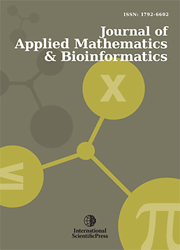# Journal of Applied Mathematics & Bioinformatics

#### MHD Couette flow of class-II in a rotating system

•[ Download ]
• Times downloaded: 1869
• Abstract

Steady MHD Couette flow of class-II of a viscous incompressible electrically conducting fluid in a rotating system is studied. Exact solution of the governing equations is obtained in closed form. Expressions for the shear stress at lower and upper plates due to primary and secondary flows and mass flow rates in primary and secondary flow directions are derived. Asymptotic behavior of the solution for velocity and induced magnetic field is analyzed for small and large values of rotation parameter K^2 and magnetic parameter M^2 to gain some physical insight into flow pattern. Heat transfer characteristics of the fluid are considered taking viscous and Joule dissipations into account. Numerical solution of energy equation and numerical values of rate of heat transfer at lower and upper plates are computed with the help of MATLAB software. The numerical values of velocity, induced magnetic field and fluid temperature are displayed graphically versus channel width variable η for various values of pertinent flow parameters whereas numerical values of the shear stress at lower and upper plates due to primary and secondary flows, mass flow rates in primary and secondary flow directions and rate of heat transfer at lower and upper plates are presented in tabular form for various values of pertinent flow parameters.ISSN: 1792-6939 (Online)
1792-6602 (Print)